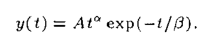# Help with curve fitting pls

Does anyone know how to fit a curve to a series of points with itk?

I currently have a cost function and a Levenberg-Marquad optimizer, but I have no idea how to get the cost function to work - I can’t find documentation anywhere.

I have a series of values (densities) at specific times, and I am trying to fit a gamma-variate function to these points. The gamma-variate function looks like this:i.e. I find the best values for A, alpha and beta.

can anyone point me in the right direction? thank you so much!

Based on the description, ITK is not really meant to solve this type of problem. I usually use Eigen for this type of problem. If you share some source code, it might ring a bell for someone.

thanks for the reply mate -

this is the cost function I’m using. I’ve copied it from an open source program here (GitHub - QIICR/DSC_Analysis: Analysis of Dynamic Susceptibility Contrast (DSC) MRI).

``````
class LMCostFunction: public itk::MultipleValuedCostFunction
{
public:
typedef LMCostFunction                    Self;
typedef itk::MultipleValuedCostFunction   Superclass;
typedef itk::SmartPointer<Self>           Pointer;
typedef itk::SmartPointer<const Self>     ConstPointer;
itkNewMacro( Self );

enum { SpaceDimension =  2 };
unsigned int RangeDimension;

enum ModelType { TOFTS_2_PARAMETER = 1 };

typedef Superclass::ParametersType              ParametersType;
typedef Superclass::DerivativeType              DerivativeType;
typedef Superclass::MeasureType                 MeasureType, ArrayType;
typedef Superclass::ParametersValueType         ValueType;

int m_ModelType;

LMCostFunction()
{
}

void SetModelType (int model) {
m_ModelType = model;
}

void SetNumberOfValues(unsigned int NumberOfValues)
{
RangeDimension = NumberOfValues;
}

//Cv = measured values
void SetCv (const float* cv, int sz) //Self signal Y
{
Cv.set_size (sz);
for (int i = 0; i < sz; ++i)
Cv[i] = cv[i];
//std::cout << "Cv: " << Cv << std::endl;
}

//Time
void SetTime (const float* cx, int sz)
{
Time.set_size (sz);
for( int i = 0; i < sz; ++i )
Time[i] = cx[i];
//std::cout << "Time: " << Time << std::endl;
}

MeasureType GetValue( const ParametersType & parameters) const
{
MeasureType measure(RangeDimension);

ValueType alpha = parameters;
ValueType beta = parameters;

ValueType deltaT = Time(1) - Time(0);

if(m_ModelType == TOFTS_2_PARAMETER)
{
measure = Cv -(); // function to 'fit' goes here I think
}

return measure;
}

MeasureType GetFittedFunction( const ParametersType & parameters) const
{
MeasureType measure(RangeDimension);

ValueType alpha = parameters;
ValueType beta = parameters;

ValueType deltaT = Time(1) - Time(0);

if(m_ModelType == TOFTS_2_PARAMETER)
{
measure = (); //function to 'fit' goes here I think
}

return measure;
}

//Not going to be used
void GetDerivative( const ParametersType & /* parameters*/,
DerivativeType  & /*derivative*/ ) const
{
}

unsigned int GetNumberOfParameters(void) const
{
if(m_ModelType == TOFTS_2_PARAMETER)
{
return 2;
}
}

unsigned int GetNumberOfValues(void) const
{
return RangeDimension;
}

protected:
virtual ~LMCostFunction(){}
private:

ArrayType Cv, Time;

ArrayType Convolution(ArrayType X, ArrayType Y) const
{
ArrayType Z;
Z = vnl_convolve(X,Y).extract(X.size(),0);
return Z;
};

ArrayType Exponential(ArrayType X) const
{
ArrayType Z;
Z.set_size(X.size());
for (unsigned int i=0; i<X.size(); i++)
{
Z[i] = exp(X(i));
}
return Z;
};

int constraintFunc(ValueType x) const
{
if (x<0||x>1)
return 1;
else
return 0;

};

};

``````

and I’m trying to use it with a Levenberg-Marquad optimizer like this:

``````itk::LevenbergMarquardtOptimizer::Pointer optimizer = itk::LevenbergMarquardtOptimizer::New();
LMCostFunction::Pointer   costFunction = LMCostFunction::New();
``````

I don’t know how to set up the cost, or ‘measure’ part correctly (I think GetValue() does this). It would have to be something like ` measure = Cv - (pow(Time, alpha)*exp(-Time/beta))` but it gives me an error that I can’t figure out how to fix.

There is an example of how to use the optimizer here:

https://itk.org/ITKExamples/src/Numerics/Optimizers/LevenbergMarquardtOptimization/Documentation.html

but no example on what the cost function has to look like.